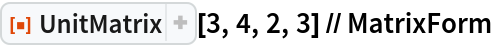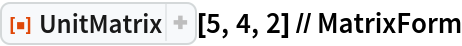Function Repository Resource:

# UnitMatrix

Get a matrix with a 1 in a selected position and 0s elsewhere

Contributed by: Dennis M Schneider
 ResourceFunction["UnitMatrix"][m,n,i,j] returns an m×n matrix whose only nonzero entry is a 1 in the (i,j)th position. ResourceFunction["UnitMatrix"][m,i,j] returns ResourceFunction["UnitMatrix"][m,m,i,j].

## Examples

### Basic Examples (2)

A 3×4 matrix whose only non-zero entry is a 1 in the (2,3) position:

 In:=Out=A 5×5 matrix whose only non-zero entry is a 1 in the (4,2) position:

 In:=Out=## Publisher

Dennis M Schneider

## Version History

• 1.0.0 – 28 August 2019